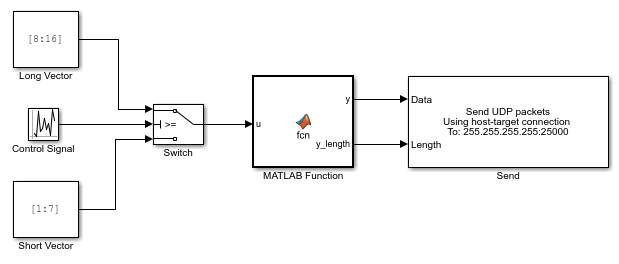## UDP and Variable-Size Signals

The Simulink® Real-Time™ UDP sublibrary does not directly support variable-size signals. The UDP Send block input port accepts only fixed-size signals.

To send variable-size signals though UDP, determine the maximum number of elements of a fixed-size input signal that you expect to connect to the block. Use the second input, `Length`, to specify the number of elements of this input signal to send through UDP.

This example configures the MATLAB Function block to accept a variable-size signal and maps that signal to a fixed-size output signal. It outputs the number of relevant elements. You can output the fixed-size output signal and number of elements to the inputs of the UDP Send block.

1. To accept a variable-size input signal, create a MATLAB Function block. MATLAB Function block inputs inherit their size, which can be variable-size.

2. In the MATLAB Function block, enter code like the following code. In this code, the maximum size of the variable-size input signal is `9`.

```function [y,y_length] = fcn(u) %#codegen y = uint8(zeros(9,1)); y_length = length(u); for a = 1:y_length y(a) = u(a); end```
3. Specify the maximum size of the output `y`.

1. Open the Symbols pane. In the Modeling tab, in the Design section, click .

2. Right-click the variable `y` and click Inspect to open the Property Inspector.

3. Enter the maximum size of the input signal into the corresponding Size property for `y`. For this example, the size value is `9`.

4. Provide a variable-size signal source to the MATLAB Function block.